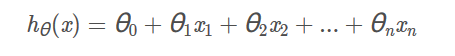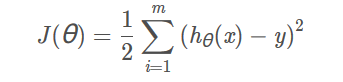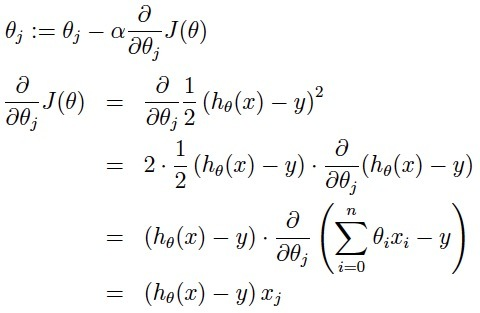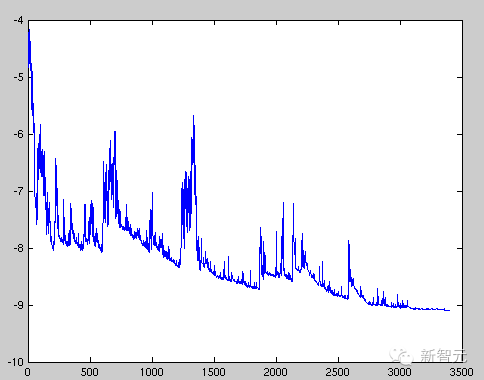• ## 随机梯度算法

千次阅读 2012-03-15 22:30:14
随机梯度下降–Stochastic Gradient Descent | 丕子 ...这时，就要乃至随机梯度下降算法了，是用来简化求最小值的计算的。先给W一个初始值，然后朝梯度方向下降，直到不能下降为止，此时即可得到对应的极小
 随机梯度下降–Stochastic Gradient Descent | 丕子  http://www.zhizhihu.com/html/y2012/3731.html
在求损失度最小值时，我们一般用到导数为0时求相应参数，但有时会导致很高的计算复杂度。这时，就要乃至随机梯度下降算法了，是用来简化求最小值的计算的。先给W一个初始值，然后朝梯度方向下降，直到不能下降为止，此时即可得到对应的极小值，但注意得到的是局部最小值，有时要对初始值进行不断修正。
记得在ML中的目标函数通常是损失+罚（$\ell(h)+\lambda f(h)$）的形式。例如ridge regression就是平方误差+$\ell_2$罚，lasso是平方误差+$\ell_1$罚，SVM是hinge loss+$\ell_2$罚。最小化这个目标函数可以等价于在$f(h)\le\delta$的限制下最小化$\ell(h)$。$\lambda$和$\delta$是一一对应的关系。实际上$f(h)\le\delta$就是定义了一个凸子空间，例如使用$\ell_2$罚时就是一个半径为$\delta$的球。所以，Online Gradient Descent可以online的解这一类目标函数，只是对于不同的罚选择不同的投影子。
下面是理论分析。记投影前的$\tilde h_{t+1} = h_t-\eta_t\nabla\ell_t(h_t)$，以及offline最优解$h^*=\arg\min_{h\in\mathcal{H}}\sum_{t=1}^T\ell_t(h)$。因为$\mathcal{H}$是凸的且$h^*$在其中，所以对$\tilde h_{t+1}$投影只会减少其与$h^*$的距离，既$\|h_{t+1}-h^*\|\le\|\tilde h_{t+1}-h^*\|$。记$\nabla_t=\nabla \ell_t(h_t)$，注意到$\displaystyle \|\tilde h_{t+1}-h^*\|^2=\|h_t-h^*\|^2+\eta_t^2\|\nabla_t\|^2-2\eta_t\langle\nabla_t,h_t-h^*\rangle.$
由于$\ell_t$是凸的，所以有$\displaystyle \ell_t(h_t)-\ell_t(h^*)\le \langle\nabla_t,h_t-h^*\rangle \le \frac{1}{2\eta_t}\big(\|h_t-h^*\|^2 - \|h_{t+1}-h^*\|^2\big) + \frac{\eta_t}{2}\|\nabla_t\|^2.$
取固定的$\eta_t=\eta$，对$t$进行累加就有$R(T)\le \frac{1}{2\eta}\|w_1-w^*\|^2+\frac{\eta}{2}\sum_{t=1}^T\|\nabla_t\|^2$。记$\mathcal{H}$的直径为$D$，且对所有$t$有$\|\nabla_t\|\le L$成立（既Lipschitz常数为$L$），再取$\eta=\frac{D}{L\sqrt{T}}$，那么$\displaystyle R(T)\le LD\sqrt{T}.$
这个bound可以通过设置变动的学习率$\eta_t$加强。

展开全文• coursera华盛顿大学机器学习专项课程第三门课分类，第七周：在线学习－随机梯度法的代码实现机器学习
• 随机梯度算法(SGDOptimizer) 随机梯度算法是神经网络中最常见的一种优化算法。主要是依据的梯度下降原理 设置要预测的函数为： 损失函数为： 则要使损失函数最小，我们可以使损失函数按照它下降速度最快的地方减小...
随机梯度算法(SGDOptimizer)
随机梯度算法是神经网络中最常见的一种优化算法。主要是依据的梯度下降原理 设置要预测的函数为：损失函数为：则要使损失函数最小，我们可以使损失函数按照它下降速度最快的地方减小，因此需要在此列出损失函数的求导公式：同时由于选用这种方法，可能会陷入局部最小值的问题，同时收敛的速度可能较慢 所以选用SGD，每次更新的时候使用一个样本进行梯度下降，所谓的随机二字，就是说我们可以随机用一个样本来表示所有的样本，来调整超参数。 因为这个样本是随机的，所以每次迭代没有办法得到一个准确的梯度，这样一来虽然每一次迭代得到的损失函数不一定是朝着全局最优方向，但是大体的方向还是朝着全局最优解的方向靠近，直到最后，得到的结果通常就会在全局最优解的附近。这种算法相比普通的梯度下降算法，收敛的速度更快，所以在一般神经网络模型训练中，随机梯度下降算法 SGD 是一种非常常见的优化算法。
展开全文• 使用随机梯度算法对线性模型进行最小二乘学习的算法流程： ①. 给θ以适当的初值。 ②. 随机选择一个训练样本（例如，选择顺序为 的训练样本（ ， ））。 ③. 对于选定的训练样本，采用使其梯度下降的方式，对参数...

使用随机梯度算法对线性模型进行最小二乘学习法的算法流程：
①. 给θ以适当的初值。
②. 随机选择一个训练样本（例如，选择顺序为 的训练样本（ ， ））。
③. 对于选定的训练样本，采用使其梯度下降的方式，对参数θ进行更新。
在这里， 是名为学习系数的无穷小正标量，表示梯度下降的步幅。 是顺序为 的训练样本相对应的训练平方误差的梯度，表示梯度下降的方向。
④．直接解 达到收敛精度为止，重复上述②、③步的计算。

转载于:https://my.oschina.net/magicalee/blog/1518232
展开全文• 基于重铸模型的MIMO非线性系统的分层扩展随机梯度法研究论文
• 借鉴https://www.sohu.com/a/131923387_473283中的一段内容：随机梯度下降算法最大的缺点就是在每次更新时可能并不会按照正确的方向进行，因此可能在优化的过程中带来扰动。如图 批处理最后得到是一个局部的最小值...
    借鉴https://www.sohu.com/a/131923387_473283中的一段内容：
随机梯度下降算法最大的缺点就是在每次更新时可能并不会按照正确的方向进行，因此可能在优化的过程中带来扰动。如图批处理最后得到是一个局部的最小值，随机处理用每一个样本更新，可能没有使用全部的数据会在另一个地方找到了局部最小值。让我用一个例子来说明这个问题：
随机梯度处理就是比如你要找一个东西，第一个村子里最可信的人对你说是黑色的，然后你往一个方向走，后来遇到第二个村子，第二村子最可信的人说是白色的，你可能有点不解往另一个方向走，然后后面的人给你的描述越来越多，最后你发现这个东西整体是黑的，局部是白的，只是每个人描述的部位不同，但最后你肯定完整的知道这个东西长什么样了，你发现大家说的都是同一个地方。同理批处理就是一次性告诉你最可信的所有的描述。
（这里要寻找的东西的路途就是最后要求的参数θ，最可信就是求极值，村子里的人就是样本，每一次走的方向就是每一次求得的参数）    所以每次给你一个样本，都会给你提供参考，找到一个参数，参数可能有错，但参考的样本越来越多，最后发现参考说的都是这个值，参数只有是这个值的时候才基本符合前面提供的所有参考。
展开全文机器学习
• （1）高斯核模型    其中为样本。可以看出，核模型的均值...（2）随机梯度下降 （3）python 代码实现 import numpy as np import matplotlib.pyplot as plt import math n=50 N=1000 x=np.linspace(-3...
• 一文通透优化算法随机梯度 随机梯度下降到牛顿 共轭梯度
• 自己编写的随机梯度下降算法，附上房价预测数据集，感兴趣的可以看看
• 一文通透优化算法：从随机梯度随机梯度下降到牛顿、共轭梯度       1 什么是梯度下降 经常在机器学习中的优化问题中看到一个算法，即梯度下降，那到底什么是梯度下降呢？ 维基百科给出的定义...
• A.data = A.data - alpha * A.grad.data b.data = b.data - alpha * b.grad.datashell
• 文章目录2.5 梯度下降介绍学习目标1 全梯度下降算法（FG）2 随机梯度下降算法（SG）3 小批量梯度下降算法（mini-batch）4 随机平均梯度下降算法（SAG）5 小结 2.5 梯度下降介绍 学习目标 知道全梯度下降算法的...机器学习
• 针对一类非均匀数据采样Hammerstein-Wiener 系统, 提出一种递阶多新息随机梯度算法. 首先基于提升技术, 推导出系统的状态空间模型, 并考虑因果约束关系, 将该模型分解成两个子系统, 利用多新息遗忘随机梯度算法辨识...
• 在机器学习领域，体梯度下降算法分为三种 - 批量梯度下降算法（BGD，...- 随机梯度下降算法（SGD，Stochastic gradient descent algorithm） - 小批量梯度下降算法（MBGD，Mini-batch gradient descent algorithm）机器学习
• 文章目录线性回归学习目标2.5 梯度下降介绍1 全梯度下降算法（FG）2 随机梯度下降算法（SG）3 小批量梯度下降算法（mini-bantch）4 随机平均梯度下降算法（SAG）5 算法比较6 梯度下降优化算法(拓展) 学习目标 ...python 神经网络 机器学习 人工智能
• 梯度下降在深度学习方面有很大的作用，但是不同情况下产生了不同的梯度下降
• 一、为什么要提出随机梯度下降算法 注意看梯度下降权值的更新方式（推导过程在上一篇文章中有）  也就是说每次更新权值都需要遍历整个数据集（注意那个求和符号），当数据量小的时候，我们还能够接受这种算法，...
• 假设函数为 ：    其损失函数为 J(θ)=1/2∑i=1m(hθ(x(i))−y(i)) 这里我们使用批量梯度下降算法，要想求θ使得J(θ)取最小，我们对于J(θ)求偏导算其梯度方向，让J(θ)...然而随机梯度下降算法不是用全体...
• 机器学习算法中回归算法有很多...梯度下降算法包含多种不同的算法，有批量梯度算法，随机梯度算法，折中梯度算法等等。对于随机梯度下降算法而言，它通过不停的判断和选择当前目标下最优的路径，从而能够在最短路径...
• 当用于训练的数据量非常大时，批量梯度下降算法变得不再适用(此时其速度会非常慢)，为解决这个问题，人们又想出了随机梯度下降算法随机梯度下降算法的核心思想并没有变，它仍是基于梯度，通过对目标函数中的参数......